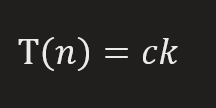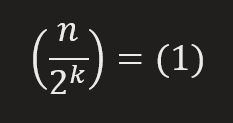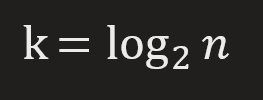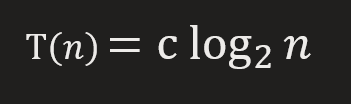# Time Complexity of binary search using Recursion Tree

What is Binary Search?

Binary Search is the shortest way of finding the element in an array (Assuming – all elements are sorted ). The advantage of sorted behavior is that we can make decisions about where to go either left or right.

For Eg –Get detailed Explanation of Binary Search here –   Binary Search – Explained

Analysis of Time complexity using Recursion Tree –For Eg – here 14 is greater than 9 (Element to be searched) so we should go on the left side, now mid is 5 since 9 is greater than 5 so we go on the right side. since 9 is mid, So element is searched.

Every time we are going to half of the array on the basis of decisions madeFor a n sized array,

The first time we are in a n sized array and then n/2 sized array and n/4 sized array and so on.

So finally we can say that element is found in N/2sized array at kth iteration

Since at the end when an element is found at kth iteration, the length of the array becomes 1.

```So, N/2k = 1
Or, N = 2k

Take log both sides
log N = k log 2
log N = k (log 2 =1)

So, time complexity = log N```

Pseudo Code:

```start
function bin_search(array,target,low,high){
if low > high return false;
calculate middle index= (low+high)/2
if middle element is equal to target element
return true

if middle element is greater than target element
bin_search(array,target,low,mid-1)

if middle element is smaller than target element
bin_search(array,target,mid+1,high)

}
end
```

Using the above pseudo-code, we can write the recurrence relation for binary search in terms of time taken by the control statements+time taken by the recursive callsWe didn’t add 2T(n/2) because for a single call, only one recursive call will be made i.e. we’ll traverse either to the left of the middle element or to the right of the middle element.

Using the above relation, the following recursion tree can be drawnIf you remember the concept from calculating upper bounds using the recursion tree method, each level will take c time to complete ( c is a constant), and we assume that there are k levels in the tree.

So the total time taken by the function would be:

```We continue this until we reach 1. So from here, we can say thatCalculating the value of k, we getSubstituting the value of k in the total time equation, we get```

To get the Big O notation, we ignore the constants and we get the time complexity of the binary search is O(logn)